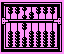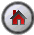Using SHAZAM as a Calculator

##Using SHAZAM as a Calculator[SHAZAM Guide home]

### Arithmetic

The `GEN1` command can be used as a calculator. For example, the command below computes and prints a sum of numbers:

 `GEN1 40+55+10 `

Results can be saved for use in subsequent calculations. This is illustrated in the next example.

 ```GEN1 REV=3000+1326 GEN1 COST=48+89.5+1200 GEN1 PROFIT=REV-COST PRINT REV COST PROFIT STOP ```

### Calculating a Sample Mean and Variance

The next commands calculate the mean, variance and standard deviation for a sample of 6 observations. Note that the calculation for the sample variance uses a divisor of 5.

 ```SAMPLE 1 6 READ X / BYVAR 23 43 26 43 13 22 STAT X STOP ```

### Calculating a Mean and Variance for 2 Different Samples

Consider 2 samples of observations. The first sample has 6 observations and the second sample has 9 observations. The commands below show how to calculate a mean and variance for each sample.

 ```SAMPLE 1 6 READ X / BYVAR 23 43 26 43 13 22 STAT X * The second sample has 9 observations SAMPLE 1 9 * Be sure to give the second variable a different name than X READ Y / BYVAR 38 58 23 678 432 23 52 2 55 STAT Y STOP ```

### Calculating the Mean Absolute Deviation

 ```SAMPLE 1 6 READ X / BYVAR 23 43 26 43 13 22 STAT X / MEAN=M GENR AD=ABS(X-M) STAT AD STOP ```

The SHAZAM output follows.

 ```|_SAMPLE 1 6 |_READ X / BYVAR 1 VARIABLES AND 6 OBSERVATIONS STARTING AT OBS 1 |_STAT X / MEAN=M NAME N MEAN ST. DEV VARIANCE MINIMUM MAXIMUM X 6 28.333 12.160 147.87 13.000 43.000 |_GENR AD=ABS(X-M) |_STAT AD NAME N MEAN ST. DEV VARIANCE MINIMUM MAXIMUM AD 6 9.7778 5.7568 33.141 2.3333 15.333 |_STOP ```

The mean absolute deviation is listed as the `MEAN` in the output from the final `STAT` command. The results show that the mean absolute deviation is 9.7778.

### Calculating Factorials and Combinations

A result from calculus is that for an integer n:(n) = (n `-` 1)!

where(n) is the gamma function. With the `GENR` or `GEN1` commands the `LGAM` function calculates the log of the gamma function. The anti-log of this function can be used to compute factorials. For example, the number 5! is calculated with the following SHAZAM commands.

 ```SAMPLE 1 1 GEN1 N=5 GEN1 FAC=EXP(LGAM(N+1)) PRINT FAC STOP ```

Now consider the problem of calculating the number of combinations of k objects chosen from n. The formula, known as the "binomial coefficient", is:An example is shown with the next SHAZAM commands.

 ```SAMPLE 1 1 GEN1 N=6 GEN1 K=3 GEN1 C=EXP(LGAM(N+1)-LGAM(K+1)-LGAM(N-K+1)) PRINT C STOP ```

The SHAZAM output follows.

 ```|_SAMPLE 1 1 |_GEN1 N=6 |_GEN1 K=3 |_GEN1 C=EXP(LGAM(N+1)-LGAM(K+1)-LGAM(N-K+1)) |_PRINT C C 20.00000 |_STOP ```

The result shows that the total number of combinations of 3 objects chosen from 6 is 20.[SHAZAM Guide home]